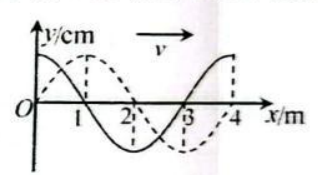$\text{A.}$ 0.1s $\text{B.}$ 0.2s $\text{C.}$ 0.4s $\text{D.}$ 0.8s